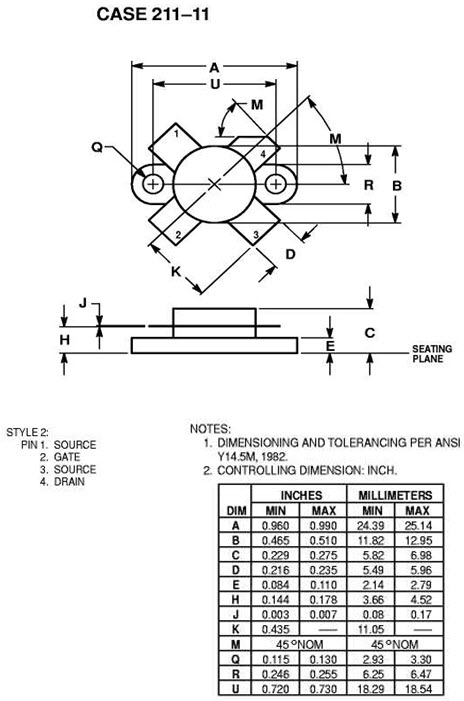# MRF151 - Silicon MOS N-Channel RF Power Transistor 150 W, up to 175 MHz, Enhancement ModeThe silicon MOS transistor is designed for professional transmitter applications in the HF frequency range.

Guaranteed Performance at 30 MHz, 50 V:

• Power Gain: 22 dB Typ
• Output Power: 150 W
• Efficiency: 40 % Typ

Guaranteed Performance at 175 MHz, 50 V:

• Power Gain: 13 dB Typ
• Output Power: 150 W
Send request
Send request

## Absolute Maximum Ratings

Drain-Source Voltage VDSS 125 VDC
Gate-Source Voltage VGS ±40 VDC
Storage Temperature Range TSTG -65 tu +150 ºC
Thermal Resistance, Junction to Case RqJC 0.6 ºC/W
Total Power Dissipation @TC=25 oC PD 300 W

## Parameters

ParameterSymbolMin.Typ.Max.Unit
Drain-Source Breakdown Voltage (ID=100.0 mA, VGS=0 V) V(BR)DSS 125 VDC
Gate-Source Leakage Current (VGS=20 V, VDS=0 V) IGSS 1.0 mADC
Zero Gate Voltage Drain Leakage Current (VDS = 50 V, VGS=0 V) IDSS 5.0 mADC
Gate Threshold Voltage (VDS = 10 V, ID = 100 mA) VGS(TH) 1.0 5.0 VDC
Forward Transconductance (VDS = 10 V, ID = 5.0 A) GFS 5.0 7.0 mhos
Input Capacitance (VDS = 50 V, VGS=0 V, f = 1 MHz) CISS 350 pF
Output Capacitance (VDS = 50 V, VGS=0 V, f = 1 MHz) COSS 220 pF
Reverse Transfer Capacitance (VDS = 50 V, VGS=0 V, f = 1 MHz) CRSS 15 pF
Power Gain (f1 = 30 MHz, f2 = 30.001 MHz, VDD = 50 V, POUT =150 W (PEP), lDQ. = 250 mA)
F=175 MHz
Gp
18
22
13
dB
Drain Efficiency (f1 = 30 MHz, f2 = 30.001 MHz, VDD = 50 V, POUT = 150 W(PEP),  lDQ. = 250 mA ) hD   45 %
Intermodulation Distortion (VDD = 50 V, Pout = 150 W (PEP),
f1 = 30 MHz, f2 = 30.001 MHz, IDQ = 250 mA)
IMD -32 dB